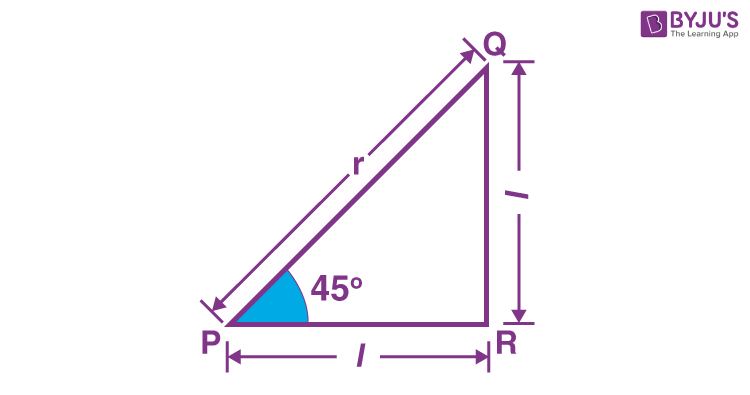# Cos 45 Degrees

The value of cos 45° is equal to 1/√2.

 Cos 45° = Cos π/4 = 1/√2

In trigonometry, the three primary ratios are sine, cosine and tangent. If the trigonometric ratio of any angle is taken for a right angled triangle, then the values depend on sides of the triangle. Cos of angle is equal to the ratio of the adjacent side and hypotenuse. Cos θ = Adjacent Side/Hypotenuse

## Cos 45° Value

The exact value of cos 45 degrees is 1/√2 (in surd form), which is also equal to sin 45 degrees. It is an irrational number, equal to 0.7071067812… in decimal form. The approximate value of cos 45 is equal to 0.7071.

 Cos 45° = 1/√2 = √2/2

Therefore, 0.7071 or 1/√2 is a value of a trigonometric function or trigonometric ratio of standard angle (45 degrees).

## Proof

Suppose we have a right-angled triangle, in which the other two angles are equal to 45 degrees. Now, if the angles of a right triangle are 45 degrees, then the adjacent sides are equal in length.Let us take the length of adjacent sides equal to ‘l’ and hypotenuse is ‘r’.

According to the Pythagoras theorem, we know that, Hypotenuse2= Perpendicular2 + Adjacent Side2

PQ2 = PR2 + QR2

⇒ r2 = l2 + l2

⇒ r = √2 l

⇒ l/r = 1/√2

Thus, Length of adjacent side/Hypotenuse = 1/√2

Therefore, we can say, Cos 45° = 1/√2

Hence, proved.

## Cos 45° and Sin 45°

We can also prove the value of cos 45° with a trigonometry approach.

As we know, sin 45° = 1/√2

Also, by trigonometric identities, we know that,

Sin2 x + cos2 x = 1

Or Cos2 x = 1 – sin2 x

Put x = 45°

Cos2 45° = 1 – sin2 45°

Put the value of sin 45° in the above equation.

Cos2 45° = 1 – (1/√2)2

Cos2 45° = 1 – ½

Cos 45° = √1/2 = 1/√2

Hence, we got the value of cos 45°.

## Cos Ratio Table

 Cos 0° 1 Cos 30° or Cos π/6 2 Cos 45° or Cos π/4 1/√2 Cos 60°or Cos π/3 √3/2 Cos 90° or Cos π/2 0 Cos 120° or Cos 2π/3 -1/2 Cos 150° or Cos 5π/6 √3/2 Cos 180° or Cos π -1 Cos 270° or Cos 3π/2 0 Cos 360°or Cos 2π 1

## Solved Examples

Question 1: Find the value of cos 45° + sin 30°

Solution: Given, cos 45° + sin 30° cos 45° + sin 30° = 1/√2 + ½ = (√2+1)/2

Question 2: Evaluate: 2 sin 60° – 4 cos 45°

Solution: Given, 2 sin 60° – 4 cos 45°

Since, sin 60° = √3/2 and cos 45° = 1/√2

Therefore, ⇒ 2 (√3/2) – 4 (1/√2)

⇒ √3 – 2√2

Question 3: Find Cos 45 + Cos 90.

Solution: Cos 45° = 1/√2 Cos 90° = 0

Therefore, Cos 45° + cos 90° = 1/√2

## Practice Questions

1. Evaluate 2 cos 45° + cos 0°
2. Find the value of sin 60° – cos 45°.
3. Tan 45° – cos 45° = ?
4. Cos 45° – ½ (Cot 45°) = ?

## Frequently Asked Questions on Cos 45 Degrees

### What is the exact value of cos 45 degrees?

The exact value of cos 45 degrees is 1/√2 or √2/2.

### Is cos 45 and sin 45 the same?

Yes, the value of cos 45 and sin 45 is the same.

### What is the value of cos 90?

The value of cos 90 is equal to 0.

### What is the sum of cos 45 and sin 45?

Cos 45 + Sin 45 = 1/√2 + 1/√2 = 2/√2 = √2

### How can you evaluate the value of cos 45?

We can use Pythagoras theorem to find the value of cos 45. Since, for a right angle, if the adjacent angles are 45 degrees, then the adjacent side and opposite side will be equal. Thus, we can find the value of cos 45 equal to 1/√2.